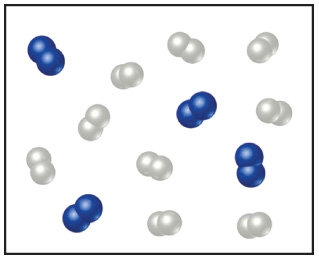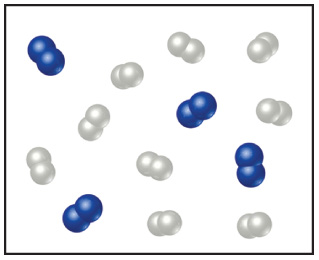# Problem: Nitrogen (N2) and hydrogen (H2) react to form ammonia (NH3). Consider the mixture of N2 and H2 shown in the accompanying diagram. The blue spheres represent N, and the white ones represent H.How many N2 molecules are left over?

###### FREE Expert Solution

Reaction:    N2 + H2 → NH3

Balanced Reaction:   N2 + 3 H2 → 2 NH3Blue spheres represent N (2 blue spheres together = N2):

4 molecules N2

White ones represent H (2 white spheres together = H2):

9 molecules N2

Calculate how much NH3 can be produced by each reactant:

From 4 molecules N2

NH3 molecules = 8 molecules

From 9 molecules H2

96% (398 ratings)###### Problem Details

Nitrogen (N2) and hydrogen (H2) react to form ammonia (NH3). Consider the mixture of N2 and H2 shown in the accompanying diagram. The blue spheres represent N, and the white ones represent H.How many N2 molecules are left over?

Frequently Asked Questions

What scientific concept do you need to know in order to solve this problem?

Our tutors have indicated that to solve this problem you will need to apply the Limiting Reagent concept. You can view video lessons to learn Limiting Reagent. Or if you need more Limiting Reagent practice, you can also practice Limiting Reagent practice problems.

What professor is this problem relevant for?

Based on our data, we think this problem is relevant for Professor Leitner's class at UNR.